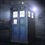# JEE AITS probs,need help!

1]If median AD of a triangle ABC makes angle 30 degrees with side BC then $$(\cot B-\cot C)^2$$ is equal to?Options:6,9,12,15.  2]$T_n=\sum_{r={2n}}^{3n-1}\dfrac{rn}{r^2+n^2},S_n=\sum_{r={2n+1}}^{3n}\dfrac{rn}{r^2+n^2}$.Then,$T_n,S_n >\ or\ < \dfrac{\ln 2}{2}$.4 years, 4 months ago

This discussion board is a place to discuss our Daily Challenges and the math and science related to those challenges. Explanations are more than just a solution — they should explain the steps and thinking strategies that you used to obtain the solution. Comments should further the discussion of math and science.

When posting on Brilliant:

• Use the emojis to react to an explanation, whether you're congratulating a job well done , or just really confused .
• Ask specific questions about the challenge or the steps in somebody's explanation. Well-posed questions can add a lot to the discussion, but posting "I don't understand!" doesn't help anyone.
• Try to contribute something new to the discussion, whether it is an extension, generalization or other idea related to the challenge.

MarkdownAppears as
*italics* or _italics_ italics
**bold** or __bold__ bold
- bulleted- list
• bulleted
• list
1. numbered2. list
1. numbered
2. list
Note: you must add a full line of space before and after lists for them to show up correctly
paragraph 1paragraph 2

paragraph 1

paragraph 2

[example link](https://brilliant.org)example link
> This is a quote
This is a quote
    # I indented these lines
# 4 spaces, and now they show
# up as a code block.

print "hello world"
# I indented these lines
# 4 spaces, and now they show
# up as a code block.

print "hello world"
MathAppears as
Remember to wrap math in $$ ... $$ or $ ... $ to ensure proper formatting.
2 \times 3 $2 \times 3$
2^{34} $2^{34}$
a_{i-1} $a_{i-1}$
\frac{2}{3} $\frac{2}{3}$
\sqrt{2} $\sqrt{2}$
\sum_{i=1}^3 $\sum_{i=1}^3$
\sin \theta $\sin \theta$
\boxed{123} $\boxed{123}$

Sort by:

The second one is based on a Jee 2008 question . I'll post the solution next week if you want me to. :)

- 4 years, 4 months ago

- 4 years, 4 months ago

Is the ans. Tn> ln2/2 and Sn<ln2/2 ?. You should see this video https://www.khanacademy.org/math/integral-calculus/indefinite-definite-integrals/riemann-sums/v/simple-riemann-approximation-using-rectangles

- 4 years, 4 months ago

Yep... Its a JEE problem based on Riemann Sums....

- 4 years, 4 months ago

But how can we use that bcoz we don't know that n tends to infinity?  And yes,best of luck!

- 4 years, 4 months ago

A definite integral is bounded between 2 summations

- 2 years, 1 month ago

Best of luck for tmmrw!

- 4 years, 4 months ago

Thanks..... :-)

- 4 years, 4 months ago

For number 1, this is what I thought of:

The way the question is worded implies that the result of $(\cot{B}-\cot{C})^2$ is always the same regardless of what the values of $m\angle{B}$ and $m\angle{C}$ are. You can use this to your advantage.

Let $m\angle{ADC}=30^\circ$, and let $m\angle{C}=90^\circ$. Now $\cot{C}=0$, and you can use 30/60/90 triangle relationships to find $\cot{B}$. I hope this helps!

- 4 years, 4 months ago